# Visual Math 4D Lite

## Graphical Calculator

3.90 TOOLS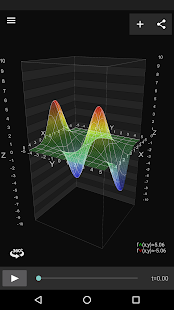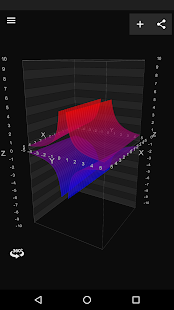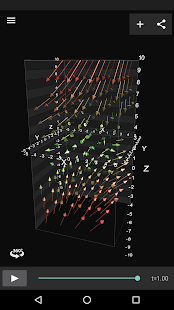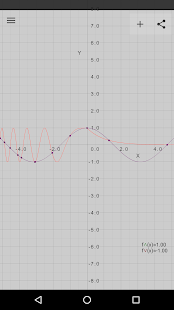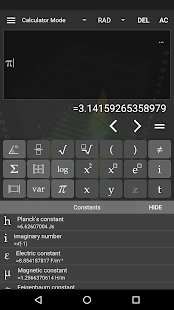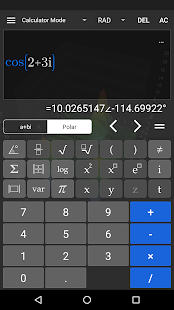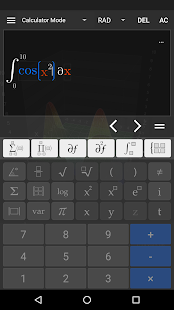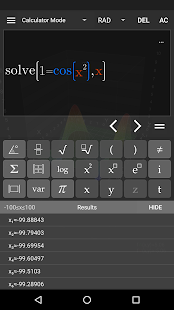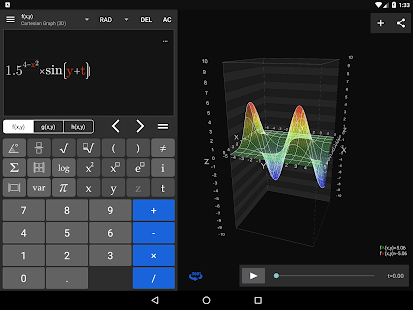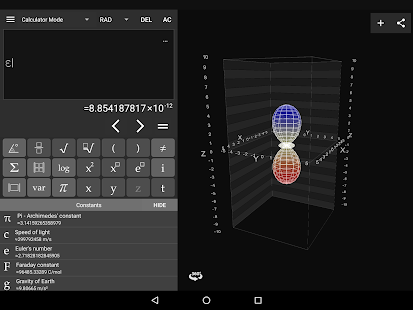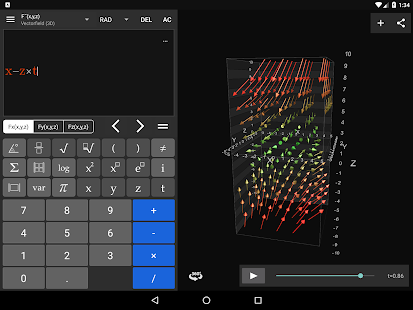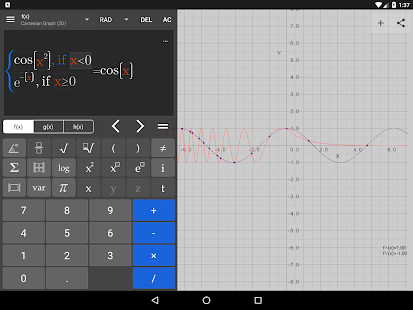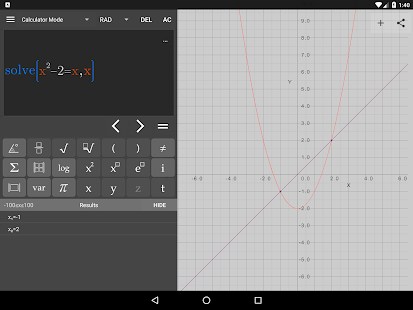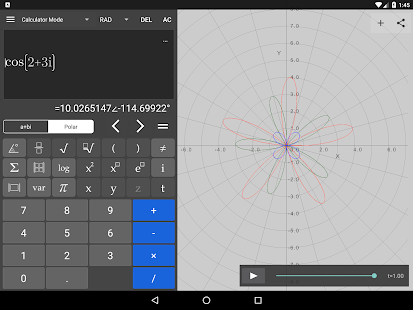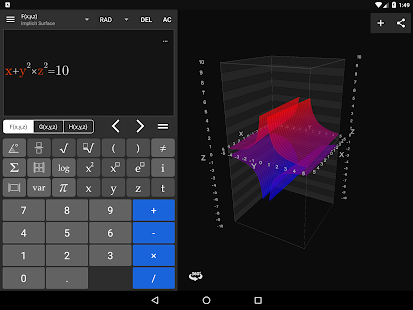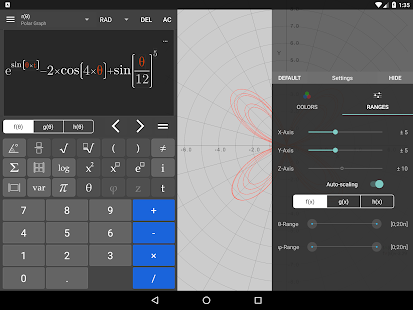Visual Math 4D Lite is a graphical calculator that allows you to visualize and solve your mathematical equations. It supports various types of equations, such as spherical, parametrical, polar and cartesian equations, which can be visualized and animated in 2D and 3D.

Features:
- solve equations
- plot cartesian functions with intersections
- plot polar- and spherical functions
- plot parametrical equations
- plot complex functions (draw real part & imaginary part)
- plot vector fields in 2D and 3D (full version)
- plot implicit equations F(x,y,z) (full version)
- complex numbers
- vectors & matrices
- truth- and value tables
- trigonometrical and hyperbolic functions
- piecewise-defined functions
- logarithmic functions
- logical & binary operators
- definite integral
- n-th derivations
- statistical functions
- physical and mathematical constants with units
- animate variables
- share content with other apps
- and a lot more...

The app is easy to use and helps students and engineers to visualize and solve their mathematical equations.

### Video

Support for Polar & Spherical equations and solving equations.

### Details and Recent Ratings

Size: 0 MB
Version: 5.1 by appnova
Updated: 23 September 2018 (1829 days ago)
Released: 21 December 2014
Installations: more than 50 000
5 Stars: 218
4 Stars: 33
3 Stars: 8
2 Stars: 8
1 Star: 75

### Similar apps

Android Game Categories
Android App Categories